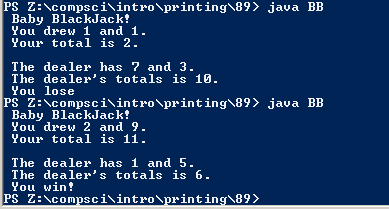# #89

## Code

```  /// Name: Josh Bautista
/// Period: 6
/// Program Name: Baby Blackjack
/// File Name: BB.java
/// Date Finished: 2/16/2015

import java.util.Random;

public class BB
{
public static void main( String[] args)
{
int n1, n2, d1, d2;
Random r = new Random();

int x = 1 + r.nextInt(10);
int x1 = 1 + r.nextInt(10);
int x2 = 1 + r.nextInt(10);
int x3 = 1 + r.nextInt(10);
System.out.println(" Baby BlackJack! ");
System.out.println(" You drew " + x + " and " + x1 + ".");
int total = x + x1;
System.out.println(" Your total is " + total + "." );
System.out.println("");
System.out.println(" The dealer has " + x2 + " and " + x3 + ".");
int total2 = x2 + x3;
System.out.println(" The dealer's totals is " + total2 + ".");
if ( total > total2 )
{
System.out.println(" You win! ");
}
else if ( total < total2 )
{
System.out.println(" You lose ");
}
}
}

```

### Picture of the output